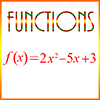# Functions – Definitions of TermsAbsolute Value: The distance a number is from 0. If x is a positive real number, then its absolute value, |x|, is x. For example, if x is 3, then |x| = |3| = 3. If x is a negative real number, then |x| = -x. For example, if x is -3, then |x| = |-3| = -(-3) = 3. Notice that both 3 and -3 are the same distance, 3, from 0.

Domain of a Function: The set of values on which the function is defined. For example, a sequence is usually defined on the non-negative integers. Therefore, the domain of a sequence is the non-negative integers.

Function: A mathematical rule that associates every element in one set – called the domain of the function – with a unique element in some other (or possibly the same) set, called the range. For example, if the function named f is defined by the polynomial 3x + 1, then both the domain and the range of the function f is the set of real numbers. Thus, if x is any real number, then the function f associates x with the value 3x + 1. This is written as f(x) = 3x + 1. This is said “f of x = 3x + 1.” Different values for x and f(x) are shown in the following table:

 x f(x) = 3x + 1 0 1 2 7 3 10 -1 -2 -2 -5

Thus, if x is 0, then f(x) is 1; if x is 2, f(x) is 7, etc. We can shorten this by saying, f(0) = 1, f(2) = 7, etc. Notice that we replace the x in the expression f(x) and in the polynomial 3x + 1 with the number 0 or the number 2, etc., so, for example, f(2) = 3·2 + 1 = 6 + 1 = 7.

Range of a Function: A set in which provides the set of values for a function. Thus, if f is a function whose domain is the set of real numbers, and f(x) = x², then the range of the function f is the non-negative real numbers.

Sequence: A series of numbers with the property that each number after the first has a particular relationship to its immediate predecessor. Example: In this sequence, 4, 7, 10, 13, … each number is 3 greater than the number preceding it. This is an example of an arithmetic sequence. The value of each number in a sequence can be determined if we know the value of the first number and the rule that determines for any given number what the next number will be.

A sequence is actually a function whose domain is usually the set of non-negative integers. Its range can vary, but is usually some subset of the real numbers.

Statistical Function: A number calculated from a set of values. The particular function is intended to identify something about the set as a whole. Commonly used functions include the mean, median, mode, range, variance, and standard deviation.